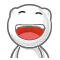Mar 2

#### 解析一个简单的分布式事务Casefelix021 @ 2018-3-2 22:41 [IT » 程序设计] 评论(1) , 引用(0) , 阅读(9107) | Via 本站原创| |1. 踩过的坑

\$dbTrans = \$db->beginTransaction();
try {
\$order = PayRequest::model()->newPayRequest(...); #在数据库中插入一条支付请求记录，状态为待支付
//其他业务改动
\$result = PaySvr::pay(\$order->id, \$order->amount); #请求PaySvr（或第三方支付通道）扣款
if (\$result['code'] == PaySvr::E_SUCCESS) {
\$order->setAsSucceeded();
} else {
\$order->setAsPending();
}
\$dbTrans->commit();
} catch (Exception \$e) {
\$dbTrans->rollback();
}

\$dbTrans = \$db->beginTransaction();
try {
\$order = PayRequest::model()->newPayRequest(...);
//其他业务改动
\$dbTrans->commit(); #先将支付请求落地
} catch (Exception \$e) {
\$dbTrans->rollback();
}

#再请求PaySvr
\$result = PaySvr::pay(\$order->id, \$order->amount);

#根据PaySvr结果修改支付请求和其他业务记录的状态
\$dbTrans = \$db->beginTransaction();
try {
if (\$result['code'] == PaySvr::E_SUCCESS) {
\$order->setAsSucceeded();
//其他业务改动
} else {
\$order->setAsPending();
//其他业务改动
}
} catch (Exception \$e) {
\$dbTrans->rollback();
}

2. 归纳总结

3. Case变种

#匹配系统
function matcher() {
\$dbTrans = \$db->beginTransaction();
try {
foreach (matchCapitalAndProject() as \$match_result) {
list(\$capital_id, \$project_id, \$amount) = \$match_result;
\$relation = Relation::model()->create(\$capital_id, \$project_id, \$amount);
\$redis->lPush(\$relation->id);
}
\$dbTrans->commit();
} catch (Exception \$e) {
\$dbTrans->rollback();
}
}

#Worker
function Worker() {
while (true) {
\$id = \$redis->brPop();
\$relation = Relation::model()->findByPk(\$id);
if (\$relation) {
BankApi::invest(\$relation->capital_id, \$relation->project_id, \$amount);
}
}
}

#匹配系统
function matcher() {
\$arr_relation = [];
\$dbTrans = \$db->beginTransaction();
try {
foreach (matchCapitalAndProject() as \$match_result) {
list(\$capital_id, \$project_id, \$amount) = \$match_result;
\$relation = Relation::model()->create(\$capital_id, \$project_id, \$amount);
\$arr_relation[] = \$relation;
}
\$dbTrans->commit();
} catch (Exception \$e) {
\$dbTrans->rollback();
}
foreach (\$arr_relation as \$relation) {
\$redis->lPush(\$relation->id);
}
}so 你們的業務技術棧是 PHP 的发表评论 打开HTML 打开UBB 打开表情 隐藏 记住我 昵称   密码   *非必须 网址   电邮   [注册]